# Class 12 Maths NCERT Solutions for Chapter 5 Continuity and Differentiability Exercise 5.8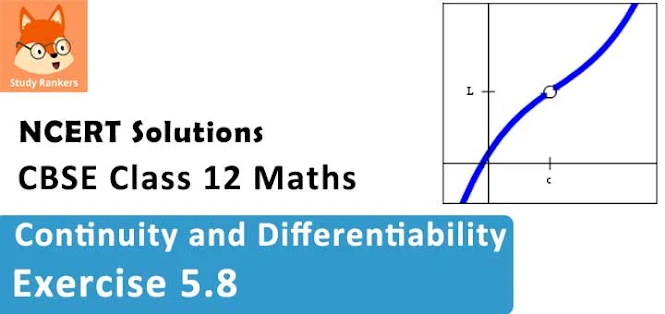### Continuity and Differentiability Exercise 5.8 Solutions

1. Verify Rolle’s theorem for the function f (x) = x2 + 2x – 8, x ∈ [– 4, 2].

Solution

The given function, f(x) = x2 + 2x – 8, being a polynomial function, is continuous in [-4, 2] and is differentiable in (-4, 2).
f(-4) = (-4)2 + 2(-4) – 8 = 16 - 8 - 8 = 0
f(2) = 22 + 2× 2 – 8 = 4 + 4 - 8 = 0
f(-4) = f(2) = 0
The value of f(x) at -4 and 2 coincides.
Rolle's theorem states that there is a point c ∈ (-4, -2) such that f'(c) = 0
f(x) = x2 + 2x – 8
⇒ f '(x) = 2x + 2
∴ f '(c) = 0
⇒ 2c + 2 = 0
⇒ c = -1, where c = -1 ∈ (-4, -2)
Hence, Rolle's Theorem is verified for the given function.

2. Examine if Rolle's Theorem is applicable to any of the following functions. Can you say some thing about the converse or Rolle's Theorem from these examples?
(i) f(x) = x for x ∈ [5, 9]
(ii) f(x) = x for x ∈ [-2, 2]
(iii) f(x) = x2 - 1 for x ∈ [1, 2]

Solution

By Rolle's Theorem, for a function f : [a, b] → R, if
(a) f is continuous on [a, b]
(b) f is differentiable on (a, b)
(c) f(a) = f(b)
then there exists some c ∈ (a, b) such that f' (c) = 0
Therefore, Rolle's Theorem is not applicable to those functions that do not satisfy any of the three conditions of the hypothesis.

(i) f(x) = x for x ∈ [5, 9]
It is evident that the given function f(x) is not continuous at every integral point.
In particular, f(x) is not continuous at x = 5 and x = 9
f(x) is not continuous in [5, 9] .
Also, f(5) =  = 5 and f(9) =  = 9
∴ f(5) ≠ f(9)
The differentiability of f in (5, 9) is checked as follows :
Let n be an integer such that n ∈ (5, 9).
The left hand limit of f at x = n is,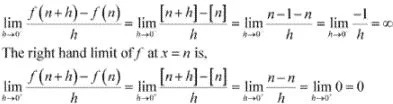Since the left and right hand limits of f at x = n are not equal, f is not differentiable at x = n
f is not differentiable in (5, 9).
It is observed that f does not satisfy all the conditions of the hypothesis of Rolle's Theorem.
Hence, Rolle's Theorem is not applicable for f(x) = [x] for x ∈ [5, 9].

(ii) f(x) = [x] for x ∈ [-2, 2]
It is evident that the given function f(x) is not continuous at every integral point.
In particular, f(x) is not continuous at x = -2 and x = 2
f(x) is not continuous in [-2, 2].
Also, f(-2) = [-2]  = -2 and f(2) =  = 2
∴ f(-2) ≠ f(2)
The differentiability of f in (-2, 2) is checked as follows.
Let n be an integer such that n ∈ (-2, 2).
The left hand limit of f at x = n is,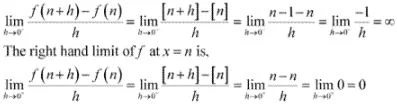Since the left and right hand limits of f at x = n are not equal, f is not differentiable at x = n
f is not differentiable in (-2, 2).
It is observed that f does not satisfy all the conditions of the hypothesis of Rolle's Theorem.
Hence, Rolle's Theorem is not applicable for f(x) = [x] for x ∈ [-2, 2] .

(iii) f(x) = x2 - 1 for x ∈ [1, 2]
It is evident that f, being a polynomial function, in continuous in [1, 2] and is differentiable in (1, 2).
f(1) = (1)2 - 1 = 0
f(2) = (2)2 - 1 = 3
f(1) ≠ f(2)
It is observed that f does not satisfy a condition of the hypothesis of Rolle's Theorem.
Hence, Rolle's Theorem is not applicable for f(x) = x2 - 1 for x ∈ [1, 2].

3. If f : [– 5, 5] → R is a differentiable function and if f ′(x) does not vanish anywhere, then prove that f (– 5) ≠ f (5).

Solution

It is given that f : [-5, 5] → R is a differentiable function .
Since every differentiable function is a continuous function, we obtain
(a) f is continuous on [-5, 5].
(b) f is differentiable on (-5, 5).
Therefore, by the Mean value Theorem, there exists c ∈ (-5, 5) such that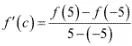⇒ 10f '(c) = f(5) - f(-5)
It is also given that f'(x) does not vanish anywhere.
∴ f '(c) ≠ 0
⇒ 10 f ' (c) ≠ 0
⇒ f(5) - f(-5) ≠ 0
⇒ f(5) ≠ f(-5)
Hence, proved.

4. Verify Mean Value Theorem, if  f(x) = x2 - 4x - 3 in the interval [a, b], where a = 1 and b = 4 .

Solution

The given function is f(x) = x2 - 4x - 3
f, being a polynomial function, is continuous in (1, 4) and is differentiable in (1, 4) whose derivative is 2x - 4.
f(1) = 12 - 4×1 - 3 = - 6, f(4) = 42 - 4 ×4 - 3 = -3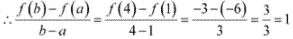Mean Value Theorem states that there is a point c ∈ (1, 4) such that f ' (c) = 1
f ' (c) = 1
⇒ 2c - 4 = 1
⇒ c = 5/2, where c = 5/2 ∈ (1, 4)
Hence, Mean value Theorem is verified for the given function.

5.Verify Mean Value Theorem, if f(x) = x3 – 5x2 – 3x in the interval [a, b], where a = 1 and b = 3. Find all c ∈ (1, 3) for which f ′(c) = 0.

Solution

The given function f is f(x) = x3 – 5x2 – 3x
f, being a polynomial function, is continuous in [1, 3] and is differentiable in (1, 3) whose derivative is  3x2 - 10x - 3 .
f(1) = 13 - 5 × 12  - 3 × 1 = -7, f(3) = 33 - 5 × 32 - 3 × 3 = -27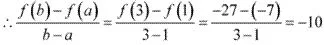Mean Value Theorem states that there exist a point c(1, 3) such that f '(c) = -10
f '(c) = -10
⇒ 3c2 - 10c - 3 = 1-0
⇒3c2 - 10c + 7 = 0
⇒ 3c2 - 3c - 7c + 7 = 0
⇒ 3c(c - 1) - 7(c - 1) = 0
⇒ (c - 1)(3c- 7) = 0
⇒ c = 1, 7/3        [where c = 7/3 ∈ (1, 3) ]
Thus, Mean Value Theorem is verified for the given function and c = 7/3 ∈ (1, 3) is the only point  for which f '(c) = 0.

6. Examine the applicability of Mean Value Theorem for all three functions given in the above exercise 2.

Solution

Mean Value Theorem states that for a function f : [a, b] → R, if
(a) f is continuous on [a, b]
(b) f is differentiable on (a, b)
then, there exists some c ∈ (a, b) such that f '(c) = [f(b) - f(a)]/(b -a)
Therefore, Mean Value Theorem is not applicable to those functions that do not satisfy any of the two conditions of the hypothesis.

(i) f(x) = [x] for x ∈ [5, 9]
It is evident that the given function f(x) is not continuous at every integral point.
In particular, f(x) is not continuous at x = 5 and x = 9
⇒ f(x) is not continuous in[5, 9].
The differentiability of f in (5, 9) in checked as follows.
Let n be an integer such that n ∈ (5, 9).
The left hand limit of f at x = n  is,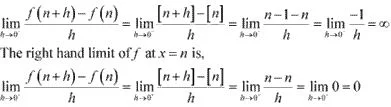Since the left and right hand limits of f at x = n are not equal, f is not differentiable at x = n
∴ f is not differentiable in (5, 9).
It is observed that f does not satisfy all the conditions of the hypothesis of Mean Value Theorem.
Hence, Mean Value Theorem is not applicable for f(x) = [x] for x ∊ [5, 9].

(ii) f(x) = [x] for x ∊ [-2, 2]
It is evident that the given function f(x) is not continuous at every integral point.
In particular, f(x) is not continuous at x = -2 and x = 2
⇒ f(x) is not continuous in [-2, 2].
The differentiability of f in (-2,2) is checked as follows .
Let n be an integer such that n ∊ (-2, 2).
The left hand limit of f at x = n is,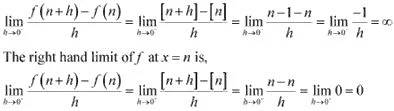Since the left and right hand limits of f at x = n are not equal, f is not differentiable at x = n
∴ f is not differentiable in (-2,2).
It is observed that f does not satisfy all the conditions of the hypothesis of Mean Value Theorem.
Hence, Mean Value Theorem is not applicable for f(x) = [x] for x ∊ [-2, 2] .

(iii) f(x) = x2 - 1 for x ∊ [1, 2]
It is evident that f, being a polynomial function, is continuous in [1, 2] and is differentiable in (1, 2).
It is observed that f satisfies all the conditions of the hypothesis of Mean Value Theorem.
Hence, Mean Value Theorem is applicable for f(x) = x2 - 1 for  x ∊ [1, 2]
It can be proved as follows.
f(1) = 12 - 1 = 0, f(2) = 22 - 1 = 3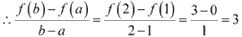f '(x) = 2x
∴ f '(c) = 3
⇒ 2c = 3
⇒ c = 3/2 = 1.5, where 1.5 ∊ [1, 2].